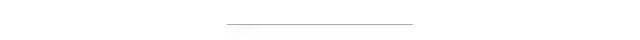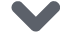# 干货 | 如何写一个更好的Python函数？Python虽然好用，但用好真的很难。

《Writing Idiomatic Python》一书的作者在Medium上发表了一篇文章，给出了6个建议。

# 什么样的函数是一个好函数？

“好”的Python函数和“差”的Python函数之间有什么差别呢？每个人都有自己的理解。基于我的理解，如果一个Python函数能够符合下面的大部分条件，我会认为它是一个“好”函数：

• 命名合理

• 单一功能

• 包括文档字符串

• 返回一个值

• 不超过50行

• 是幂等函数或纯函数

# 命名

def get knn(from_df):

• “get”这个词是无关紧要的。对于大多数命名比较好的函数来说，很明显有一些东西会从函数中返回，它的名字将反映这一点。

• from_df也不是必要的。如果没有明确的参数名称，函数的文档字符串或类型注释会描述参数的类型。

def k_nearest_neighbors(dataframe):

# 单一功能

def calculate_and print_stats(list_of_numbers):
sum = sum(list_of_numbers)
mean = statistics.mean(list_of_numbers)
median = statistics.median(list_of_numbers)
mode = statistics.mode(list_of_numbers)
print(‘—————–Stats—————–‘
print(‘SUM: {}’.format(sum) print(‘MEAN: {}’.format(mean)
print(‘MEDIAN: {}’.format(median)
print(‘MODE: {}’.format(mode)

# 文档字符串（Docstrings）

• 每个函数都需要有一个文档字符串

• 使用适当的语法和标点符号；用完整的句子写

• 首先对函数的作用进行一句话的总结

• 使用说明性语言而不是描述性语言

# 返回值

❯ python3
Python 3.7.0 (default, Jul 23 201820:22:55)
[Clang 9.1.0 (clang-902.0.39.2)] on darwin
…   print(a + b)

3
>>> b
>>> b is None
True

with open(‘foo.txt’‘r’as input_file:
for line in input_file:
if line.strip().lower().endswith(‘cat’):
# … do something useful with these lines

if line.strip().lower().endswith(‘cat’):这一行能够工作，是因为每个字符串方法( strip ( )、lower ( )、end swith ( ) )都返回一个字符串作为调用函数的结果。

# 幂等和函数纯度

def add_three(number):    """Return *number* + 3."""    return number + 3

“””Return 3 + the number entered by the user.”””
number = int(input(‘Enter a number: ‘))
return number + 3

## 什么是纯函数？

“””Return *number* + 3.”””
print(f’Returning {number + 3})
return number + 3
def num_calls():
“””Return the number of times *add_three* was called.”””

https://hackernoon.com/write-better-python-functions-c3a9a36382a6点击下方 |  | 了解更多